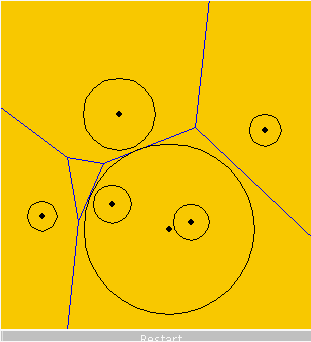Prev Next
Reminder: Power DiagramRecall earlier discussion of weighted Voronoi diagrams These are also called power diagrams Very similar to Voronoi diagram, except each point is also given a weight Cell of an input point p is then the set of points that have a smaller weighted distance to p than to any other point